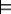#### 6.1.3.1 Semantics of Conditional Probability

Evidence e will rule out all possible worlds that are incompatible with e. Like the definition of logical consequence, the given formula e selects the possible worlds in which e is true. Evidence e induces a new measure, µe, over possible worlds where all worlds in which e is false have measure 0, and the remaining worlds are normalized so that the sum of the measures of the worlds is 1.

Here we go back to basic principles to define conditional probability. This basic definition is often useful when faced with unusual cases.

The definition of the measure follows from two intuitive properties:

• If S is a set of possible worlds, all of which have e true, define µe(S) = c×µ(S) for some constant c (which we derive below).
• If S is a set of worlds, all of which have e false, define µe(S)=0.

We want µe to be a probability measure, so if Ω is the set of all possible worlds, µe(Ω)=1. Thus, 1=µe(Ω)=µe({ω:ωe})+µe({ω:ωe})=c×µ({ω:ωe})+0=c×P(e). Therefore, c=1/P(e).

The conditional probability of formula h given evidence e is the measure, using µe, of the possible worlds in which h is true. That is,

 P(h|e) = µe({ ω:ωh} ) = µe({ ω:ωh∧e} ) + µe({ ω:ωh∧¬e} ) = µ({ ω:ωh∧e})/P(e)+0 = P(h∧e)/P(e).

The last form above is usually given as the definition of conditional probability.

For the rest of this chapter, assume that, if e is the evidence, P(e)>0. We do not consider the problem of conditioning on propositions with zero probability (i.e., on sets of worlds with measure zero).

A conditional probability distribution, written P(X|Y) where X and Y are variables or sets of variables, is a function of the variables: given a value x∈dom(X) for X and a value y∈dom(Y) for Y, it gives the value P(X=x|Y=y), where the latter is the conditional probability of the propositions.

Background Knowledge and Observation

The difference between background knowledge and observation was described in Section 5.3.1. When we use reasoning under uncertainty, the background model is described in terms of a probabilistic model, and the observations form evidence that must be conditioned on.

Within probability, there are two ways to state that a is true:

• The first is to state that the probability of a is 1 by writing P(a)=1.
• The second is to condition on a, which involves using a on the right-hand side of the conditional bar, as in P(·| a).

The first method states that a is true in all possible worlds. The second says that the agent is only interested in worlds where a happens to be true.

Suppose an agent was told about a particular animal:

 P(flies|bird) = 0.8, P(bird|emu) = 1.0, P(flies|emu) = 0.001.

If it determines the animal is an emu, it cannot add the statement P(emu)=1. No probability distribution satisfies these four assertions. If emu were true in all possible worlds, it would not be the case that in 0.8 of the possible worlds, the individual flies. The agent, instead, must condition on the fact that the individual is an emu.

To build a probability model, a knowledge base designer must take some knowledge into consideration and build a probability model based on this knowledge. All subsequent knowledge acquired must be treated as observations that are conditioned on.

Suppose the agent's observations at some time are given by the proposition k. The agent's subsequent belief states can be modeled by either of the following:

• construct a probability theory, based on a measure µ, for the agent's belief before it had observed k and then condition on the evidence k conjoined with the subsequent evidence e, or
• construct a probability theory, based on a measure µk, which models the agent's beliefs after observing k, and then condition on subsequent evidence e.

All subsequent probabilities will be identical no matter which construction was used. Building µk directly is sometimes easier because the model does not have to cover the cases of when k is false. Sometimes, however, it is easier to build µ and condition on k.

What is important is that there is a coherent stage where the probability model is reasonable and where every subsequent observation is conditioned on.

The definition of conditional probability lets us decompose a conjunction into a product of conditional probabilities:

Proposition 6.3: (Chain rule) Conditional probabilities can be used to decompose conjunctions. For any propositions α1,...,αn:
 P(α1∧α2∧...∧αn) = P(α1)× P(α2|α1)× P(α3|α1 ∧α2)× ... P(αn|α1∧···∧αn-1) = ∏i=1n P(αi|α1∧···∧αi-1),

where the right-hand side is assumed to be zero if any of the products are zero (even if some of them are undefined).

Note that any theorem about unconditional probabilities is a theorem about conditional probabilities if you add the same evidence to each probability. This is because the conditional probability measure is another probability measure.